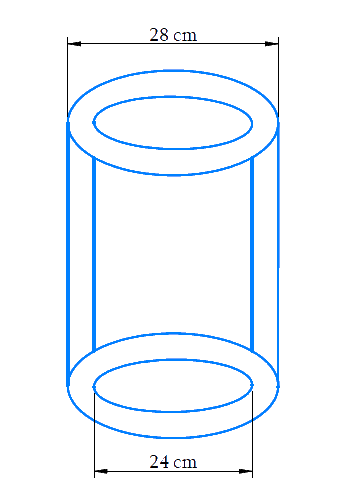# Ex.13.6 Q2 Surface Areas and Volumes Solution - NCERT Maths Class 9

Go back to  'Ex.13.6'

## Question

The inner diameter of a cylindrical wooden pipe is $$24 \;cm$$ and its outer diameter is $$28 \;cm$$. The length of the pipe is $$35\ cm$$. Find the mass of the pipe, if \begin{align}1\,\,c{m^3} \end{align} of wood has a mass of $$0.6\; g$$.

Video Solution
Surface-Areas-And-Volumes
Ex exercise-13-6 | Question 2

## Text Solution

Reasoning:

Since the cylindrical wooden pipe is made up of two concentric circles at the top and bottom, we have to find the volume of both the cylinders.

DiagramWhat is known?

Diameter of the inner and outer cylinder. Length of the cylinder.

What is unknown?

Mass of the pipe if  $$1 \mathrm{cm}^{3}$$ of pipe has mass $$6\; g$$.

Steps:

Volume of the outer cylinder $$V_{1}=\pi R^{2} h$$

Outer diameter $$(2R) = 28\; cm$$

Outer radius \begin{align}(R)=\frac{28}{2}=14 \mathrm{cm}\end{align}

Height $$(h) = 35\ cm$$

Volume of outer cylinder

\begin{align}V_{1}&=\frac{22}{7} \times 14 \times 14 \times 35\\&=21560 \;\rm{cm}^{3}\end{align}

Volume of inner cylinder $$V_{2}=\pi r^{2} h$$

Inner diameter \begin{align} \left( {2r} \right) = 24\,cm. \end{align}

Inner radius \begin{align} (r) = \frac{{24}}{2} = 12\,cm \end{align}

Height $$(h) = 35\ cm$$

Volume of outer cylinder

\begin{align} {V_2} &= \frac{{22}}{7} \times 12 \times 12 \times 35 \\&= 15840\,c{m^3} \end{align}

Volume of the wood used $$=$$ Outer volume $$-$$ Inner volume

\begin{align} &= 21560 - 15840 \\ & = 5720\;{\rm{cm}}^{\rm{3}} \\ \end{align}

Mass of  $$\mathrm{1cm}^{3} \text { pipe }=0.6 \mathrm{g}$$

Therefore,

Mass of $$5720 \,{cm}^{3}$$ pipe

\begin{align} &={ 0}{\rm{.6 \times 5720}}\\ &= 3432\,\,g\\&\quad\rm OR\\ &= 3.432\,\,kg \end{align}

Answer: Mass of the pipe $$= 3.432\ kg$$.

Learn from the best math teachers and top your exams

• Live one on one classroom and doubt clearing
• Practice worksheets in and after class for conceptual clarity
• Personalized curriculum to keep up with school